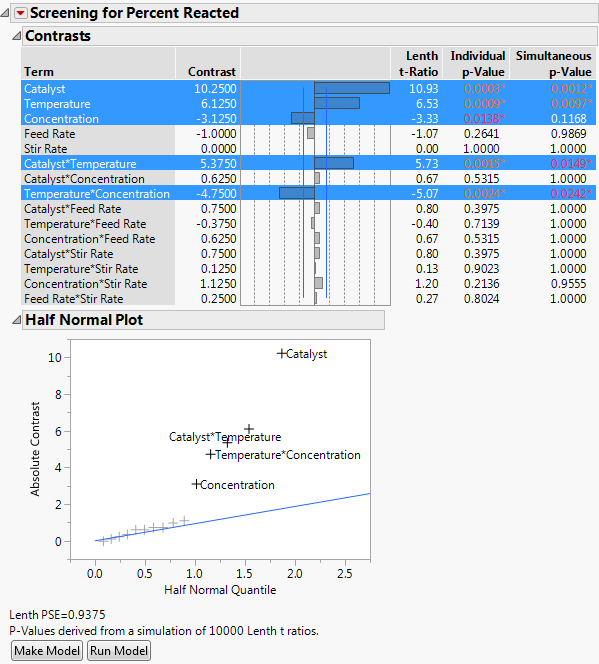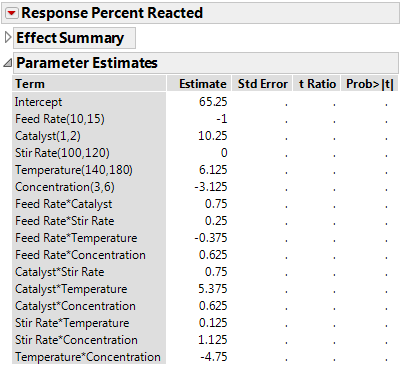Design of Experiments Guide > The Fit Two Level Screening Platform > Example of Fit Two Level Screening Platform
Publication date: 05/24/2021

# Example of Fit Two Level Screening Platform

The Reactor Half Fraction.jmp sample data table contains the results of an experiment derived from a design discussed in Box et al. (1978). You are interested in identifying significant effects in a model that contains main effects and two-way interactions. This example uses a model with fifteen parameters for a design with sixteen runs. The example illustrates the analysis both with the Fit Two Level Screening Platform and the Fit Model Platform.

#### Fit Two Level Screening

1. Select Help > Sample Data Library and open Reactor Half Fraction.jmp.

2. Select DOE > Classical > Two Level Screening > Fit Two Level Screening.

3. Select Percent Reacted and click Y.

4. Select Feed Rate through Concentration, click X, and then click OK.

Note: The Fit Two Level Screening Platform automatically constructs a model with interaction terms. This is in contrast to the Fit Model Platform, where you manually specify the interactions that you want to include in your model.

Figure 10.2 Reactor Half Fraction.jmp Fit Two Level Screening Design ReportNote the following features of the Screening report:

The effects that have an individual p-value less than 0.10 are selected.

A t-ratio is calculated using Lenth’s PSE (pseudo-standard error). The Lenth PSE value is shown below the Half Normal Plot.

Both individual and simultaneous p-values are shown. Those that are less than 0.05 are shown with an asterisk.

The Half Normal Plot enables you to quickly examine the effects. The effects that are initially selected in the effects list are also labeled in this plot.

In this example, Catalyst, Temperature, and Concentration, along with two of their two-factor interactions, are selected. Alternatively, you can fit the same model in the Fit Model Platform.

#### Fit Model

1. From the Reactor Half Fraction.jmp table, select Analyze > Fit Model.

2. Select Percent Reacted, click Y.

3. Select Feed Rate through Concentration, and then select Macros > Factorial to Degree.

4. Click Run.

5. Open the Parameter Estimates outline.

Figure 10.3 Saturated Reactor Half Fraction.jmp Design Parameter EstimatesSince there are 16 observations and 16 model terms, there are not enough observations to estimate an error term. Without an estimate of error, it is not possible to conduct standard tests. Parameter estimates are provided, but because there are no degrees of freedom for error, standard errors, t-ratios, and p-values are all missing. This illustrates the strength of the Fit Two Level Screening platform for getting the most information out of a screening design.